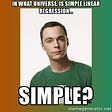# Naive Bayes in Machine Learning

1. Introduction:
• P(c|x) is the posterior probability of class (outcome) given predictor (feature).
• P(c) is the prior probability of class.
• P(x|c) is the likelihood which is the probability of the predictor given class.
• P(x) is the prior probability of predictor.
• Real-time prediction: Naive Bayes is an eager learning classifier and is quite fast in its execution. Thus, it could be used for making predictions in real-time.
• Multi-class prediction: The Naive Bayes algorithm is also well-known for multi-class prediction, or classifying instances into one of several classes.
• Text classification or spam filtering or sentiment analysis: When used to classify text, a Naive Bayes classifier often achieves a higher success rate than other algorithms due to its ability to perform well on multi-class problems while assuming independence. As a result, it is widely used in spam filtering (identifying spam email) and sentiment analysis (e.g. in social media, to identify positive and negative customer sentiments).
• Recommendation Systems: A Naive Bayes Classifier can be used together with Collaborative Filtering to build a Recommendation System that could filter through new information and predict whether a user would like a given resource or not.
• It is easy and fast to predict the class of test data set. It also performs well in multi-class prediction
• When the assumption of independence holds, a Naive Bayes classifier performs better compared to other models like logistic regression, and it needs less training data.
• It performs well in the case of categorical input variables compared to the numerical variables. For numerical variables, the normal distribution is assumed (bell curve, which is a strong assumption).
• If the categorical variable has a category (in the test data set), which was not observed in the training data set, then the model will assign a 0 probability and will be unable to make a prediction. This is often known as “Zero Frequency”. To solve this, we can use the smoothing technique like Laplace estimation.
• On the other side, naive Bayes is also known as a bad estimator, so the probability outputs from predict_proba are not to be taken too seriously.
• Another limitation of Naive Bayes is the assumption of independent predictors. In real life, it is almost impossible that we get a set of predictors which are completely independent.
• Gaussian: It is used in classification, and it assumes that features follow a normal distribution. With the iris dataset (which follows Gaussian distribution) GaussianNB model is as follows:
• Multinomial: The multinomial Naive Bayes classifier is suitable for classification with discrete features (e.g., word counts for text classification). The multinomial distribution normally requires integer feature counts. Below is an example of Multinomial Naive Bayes with the MNIST digit dataset. Each sample (belonging to 10 classes) is an 8×8 image encoded as an unsigned integer (0–255):
• Bernoulli: If X is random variable Bernoulli-distributed, it can assume only two values (Example: 0 and 1) and their probability is:
• If continuous features do not have a normal distribution, we should use log transformation or different methods to convert them into the normal distribution.
• Remove correlated features, as the highly correlated features are voted twice in the model, and it can lead to overinflating importance.
• Naive Bayes classifiers have limited options for parameter tuning like α=1 for smoothing, fit_prior=[True|False] to learn class prior probabilities or not and some other options. It is recommended to focus on pre-processing of data and feature selection.
• You might think to apply some classifier combination techniques like ensembling, bagging, and boosting, but these methods would not help. Actually, ensembling, boosting, bagging won’t help since their purpose is to reduce variance. Naive Bayes has no variance to minimize.

--

--

--

## More from Heena Sharma

Love podcasts or audiobooks? Learn on the go with our new app.

## Countering Internal Covariate Shift with Batch Normalization## [CNIT 581-SDR/Spring 2019] Week 17 — Final Blog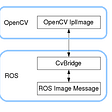## Lecture Notes in Deep Learning: Activations, Convolutions, and Pooling — Part 2## Artificial Neural Networks -2, explained differently to my son## Dependency Parser or how to find syntactic neighbours of a word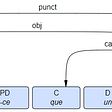## Building a simple Neural Network with Keras and Tensorflow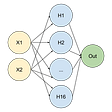## A Simple Outline of Reinforcement Learning## Building a Feature Store to reduce the time to production of ML models## Explaining Linear Regression## Gradient Descent in Machine Learning: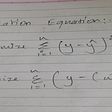## Simple Linear Regression Simplified